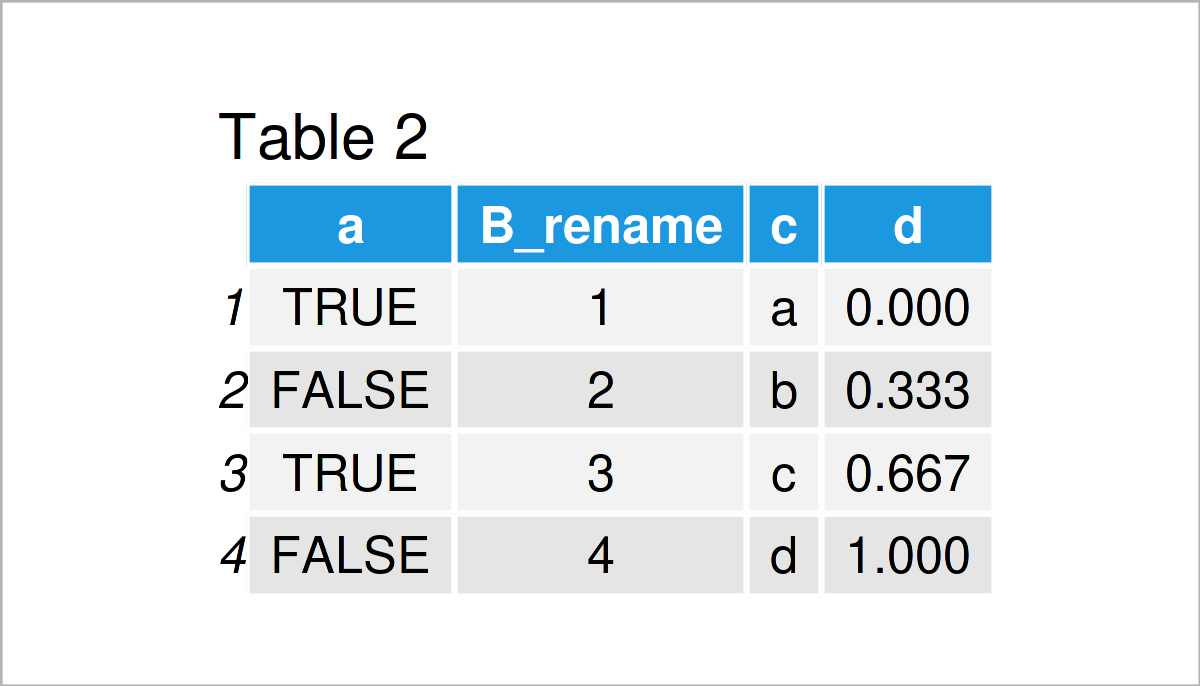# Rename Columns of data.table in R (2 Examples)

In this post you’ll learn how to change the column names of a data.table in the R programming language.

Here’s how to do it:

## Example Data & Add-On Packages

To use the functions of the data.table package (github page here & our introduction on Statistics Globe here), we first have to install and load data.table to R:

```install.packages("data.table") # Install data.table package library("data.table") # Load data.table```

As a next step, I’ll also have to create some data that we can use in the examples below:

```ex_dt <- data.table(a = c(TRUE, FALSE, TRUE, FALSE), b = 1:4, c = letters[1:4], d = seq(0, 1, length.out = 4)) # Create data.table ex_dt # Print data```Have a look at the previous table. It shows that our example data is made up of four rows and four columns. We explicitly chose the column names of the data table in the code lines above.

## Example 1: Rename a Column in a data.table

This section demonstrates how to rename the columns of the example data.table.

```ex_dt_2 <- data.table::copy(ex_dt) # Duplicate the data colnames(ex_dt_2) # Print column names #  "a" "b" "c" "d" colnames(ex_dt_2) <- "B_rename" # Rename second column name ex_dt_2```In Table 2 you can see that we have created a second data.table with a new column name by running the previously shown R code. That is, we indexed column name number two and assigned a new name to the column.

## Example 2: Rename a Column in a data.table Without Indexing the Column

In this section, I’ll demonstrate how to change the name of a column without indexing it. Sometimes, our dataset consists of many columns and we may not be sure which is the number of the column that we want to rename.

```ex_dt_3 <- data.table::copy(ex_dt) # Duplicate the data colnames(ex_dt_3) # Print column names #  "a" "b" "c" "d" colnames(ex_dt_3)[colnames(ex_dt_3) == "c"] <- "C_rename" # Rename the column which was names "c" ex_dt_3```As shown in Table 3, the previous R syntax has created a data.table, where the name of the column, which was previously named c, was changed to C_rename.

## Video & Further Resources

Have a look at the following video on my YouTube channel. I illustrate the R programming codes of this page in the video.

In addition, you may want to have a look at the other articles on my website.

Summary: In this article, you have learned how to edit the column names of a data.table in R programming. Don’t hesitate to please let me know in the comments, if you have any additional questions.

This page was created in collaboration with Anna-Lena Wölwer. Have a look at Anna-Lena’s author page to get further information about her academic background and the other articles she has written for Statistics Globe.

Subscribe to the Statistics Globe Newsletter